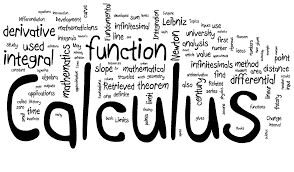•AP Calculus class code:  q4kmdvm

Honors Calculus class code: zvgsu4w

COURSE OVERVIEW

In Calculus our primary textbook is Calculus of a Single Variable, 10 t h  Edition by Larson.  Other material, test questions, and examples are taken from numerous textbooks, AP review books, and online websites, including, but not limited to the AP Central website. Throughout the year, students are exposed to, and expected to discuss and solve problems graphically, analytically, verbally, and numerically. Students will become proficient at using the graphing calculator through daily practice and assignments.  It is the objective of this course to adequately prepare students for the Advanced Placement exam while providing the students a college level education that will prepare them to begin college with a higher level of mathematical understanding.

COURSE PLANNER

Preparation for Calculus

Limits and Their Properties

Independent work over the summer. Collected and graded upon returning to school.

Differentiation

· Tangent line vs. secant line

· Definition of the derivative

· Continuity vs. Differentiability

· Existence of a derivative (absolute values)

· Basic differentiation rules

o Product, quotient, chain rules

o Trigonometric functions

· Higher order derivatives

· The graph of f(x) and f1(x)

· Rates of change

· Vertical motion

· Implicit Differentiation

· Related Rates and their applications

Graphing f(x) and f’(x)

activity

Existence of derivative and limits activity

Logarithmic and Exponential Functions

· Simplification using natural and exponential functions

· Differentiation and the natural log and exponential functions

Applications of Derivatives

· Extrema on an interval

o Local and global extrema

o Critical Numbers

o Application of Rolle’s theorem, the mean value theorem, and the intermediate value theorem

· Analyzing the graph of f(x) and f(x)

o Sketching either graph

o Analyzing the graphs

o Reading the graphs through tables

· The first derivative test

o Intervals of increasing/decreasing

· Applications of the first derivative test

· The second derivative test

o Points of inflection

o Concavity

o Maximum/Minimum Values

o Applications of the second derivative test

· Curve sketching

o Using the first and second derivative tests

o Limits at infinity

o Asymptotes

o Extreme values

· Optimization and Modeling

· Differentials

Integration

· Antiderivatives and indefinite integration

· General vs. Particular solution

· Slope fields

· Approximations of area

o Riemann Sums

o Trapezoidal Rule

· Horizontal Motion

· The Fundamental Theorem of Calculus

o Properties of the definite integral

· Calculating distance

· The Second Fundamental Theorem of Calculus

· Integration by substitution

Logarithmic and Exponential Functions

· Inverse Functions

· Integration and the natural logarithmic and exponential functions

· Differential equations and exponential growth and decay

· Separation of variables

· Inverse Trigonometric functions and their derivative and integrals

Applications of Integration

· Area between 2 curves

· Methods of computing volumes

o Disc Method

o Shell Method

Grades will be based on major tests, quizzes, homework, and class participation.
Tests – 45%
Quizzes – 35%

Homework and Class Participation – 10% each

TESTS AND QUIZZES

Numerical grades are used to grade all tests and quizzes.  The purpose of this is so that you can keep up with the information and know if you understand what is going on in class.  This also helps you when there are chapter tests because you have already studied most of the information for your quizzes.  Tests and quizzes will always be announced in advance. Cheating will result in an automatic failure.  Your lowest quiz grade will be dropped.

EXTRA HELP

If you need extra help, please make arrangements with me in class.  Ask me for help immediately if there is material you do not understand.  Please do not wait until the day before a major test to seek help!